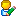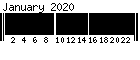## Mail System Error - Returned Mail

This Message was undeliverable due to the following reason:=E3=81=93=E3=81=AE=E3=83=A1=E3=83=83=E3=82=BB=E3=83=BC=E3=82=B8=E3=81=AF==E3=80=81=E4=BB=A5=E4=B8=8B=E3=81=AE=E7=90=86=E7=94=B1=E3=81=AB=E3=82=88==E3=81=A3=E3=81=A6=E9=85=8D=E9=80=81=E3=81=A7=E3=81=8D=E3=81=BE=E3=81=9B==E3=82=93=E3=81=A7=E3=81=97=E3=81=9F:

Your message is larger than the destination computer is willing toaccept, so it was returned. The error message below indicates thesize of your message and the maximum size allowed by the receivingE-mail system. You may be able to split your message into severalsmaller pieces and have them delivered separately.

Size of this message: 7610438 bytesServer maximum size: 3145728 bytes

The following recipients did not receive this message:

— posted by Nob at 09:58 amComment 

## IHF CUP ヘリ大会 兼 技能証検定会

11月17日(日)IHF CUP ヘリ大会

2019年11月17日　開催

IHF CUP ヘリ大会 兼 技能証検定会

※各種目は日本模型航空連盟ラジオコントロール・ヘリコプター技能証規程に準ずる。

[初級クラス] 技能証規定A級（定員10名）

[中級クラス] 技能証規定B級（定員10名）

[上級クラス] 技能証規定C級（定員10名）

[F3Cクラス] F3C 2020年度 新パターン（定員25名予定）

F3Cクラス　３000円

※申込期限前でも、ご入金順に定員数までで締め切りますのであらかじめご了承ください。申し込みの返信メールにて振り込み口座を連絡いたします。

— posted by Nob at 03:29 pmComment 

1 2 3 4
5 6 7 8 9 10 11
12 13 14 15 16 17 18
19 20 21 22 23 24 25
26 27 28 29 30 31

Created in 0.2930 sec.
T:Y:ALL:Online: